HomeTallyPrimeAccountingInterest Calculation
Explore Categories

# Interest Calculation in TallyPrime

Interest is a legitimate return on money invested and chargeable in the business world on loans and also on delayed payments. Interest can be calculated on the basis of Simple or Compound Interest. With TallyPrime you can make the required configurations and view the reports on interest calculated based on these configurations. In TallyPrime, the calculation of interest will be based on outstanding receivables or payables.

## Enable Interest Calculation in TallyPrime

1. Press F11 (Features) > set Enable Interest Calculation to Yes.
If you do not see this option:
2. Set Show all features to Yes.
2. Press Ctrl+A to save the Company Features Alteration screen.

## Interest Calculation Using Simple Parameters

Interest calculation on outstanding balances is allowed for any ledger account. Configure the interest rate, and style of calculation in the ledger master, to start calculating interest on outstanding amounts. Interest on outstanding balances can also be calculated transaction-by-transaction.

1. Gateway of Tally > Alter > Ledger > select the bank ledger > and press Enter.
Alternatively, press Alt+G (Go To) > Alter Master > Ledger > select the bank ledger > and press Enter.
2. Set the option Activate interest calculation to Yes. The Interest Parameters screen appears.
3. In the Calculate Interest Based On field select the Date Type, as required.
The option to calculate interest based on either Bank reconciliation date or voucher date will be displayed for ledgers grouped under Bank Accounts, Bank OCC A/c and Bank OD A/c. The interest calculated on:
• Voucher Date will be displayed in the Ledger Interest report.
• Bank/Reco Date will be displayed in Bank Reconciled Transactions report.
4. Set Include transaction date for interest calculation, as needed. This can be enabled For Amounts added and/or For Amounts Deducted.
5. Enter the Rate of interest.
6. Select the Interest Style, as required. The rate of interest will be calculated on the basis of the interest style applied.
`Note: If the interest style selected is 365-day Year, then the interest will be calculated for 365 days irrespective of the number of days in the calendar year. If Calendar Year is selected as the interest style, then the rate of interest will be calculated on 365 days or 366 days, depending on the number of days as per the calendar.`
7. Select the required details in as needed.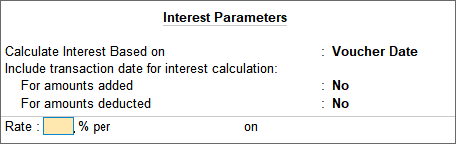8. Press Ctrl+A to save.

## Interest Calculation Transaction-by-Transaction

Calculation of interest can be done bill-by-bill for accounts grouped under Sundry Debtors and Sundry Creditors.

1. In the party ledger, set the option Activate interest calculation to Yes. The Interest Parameters screen appears.
2. Set the option Calculate Interest Transaction-by-transaction to Yes.
3. The option Include transaction date for interest calculations: For amounts added, For amounts deducted is provided in the Interest Parameters screen. This option allows you to exclude or include the transaction date for amounts added and deducted. For example, for a sundry debtor ledger, there was an invoice raised for Rs. 50,000 on 1st of April 2020, against which an amount of Rs. 20,000 was received on the same day. Now you may want to include the invoice amount and exclude the received amount, for the purpose of calculating interest on 1st April 2020.
4. Set the option Override Parameters for each Transaction to Yes, to change the parameters while recording vouchers.
5. Enter the Rate of interest.
6. Select the Interest Style, as required. The rate of interest will be calculated on the basis of the interest style applied.7. To exclude or include the transactions, you can use the options shown below:
 For amounts deducted For amounts added Yes No Yes Includes both the additions and deductions on the transaction date to the amount. Interest is calculated after considering the amount of the transaction date. The additions done on the transaction date will be considered from the next day onwards, whereas, the deductions are considered on the transaction date for calculating interest. No The additions are included on the transaction date, and the deductions are excluded from calculating interest on the transaction date. The interest on the deductions is calculated on the next day. Excludes both the additions and deductions on the transaction date. The interest is calculated on the net amount from the next day.
`Note: The terms additions and deductions are used in relation to the opening balance (either zero or any value). When any amount is added to opening balance, it should be treated as addition, and when any amount is reduced from opening balance, it should be treated as deduction. In other words, the ‘interest calculation for the day’ means opening balance + additions – deductions, which is the closing balance of the day.`

## Calculate Interest Using Advanced Parameters

The advanced parameters are used when interest rates change periodically. Using the advanced parameters, you can configure the number of days, balance type, and applicable from date that are to be considered for calculating interest.

In this section

### Set advanced interest parameters for a party

1. In the Interest Parameters screen of the party ledger, press F12 (Configure) and set Use advanced interest parameters to Yes.
2. Set the option Calculate Interest Transaction-by-Transaction to Yes, to calculate interest for each transaction.
3. Set the option Override Parameters for each Transaction to Yes to display the Interest Parameters screen while recording the transaction. The defined parameters can be modified during voucher entry.
4. Set the option Override advanced parameters to Yes.
5. Set Include transaction date for interest calculation, as needed. This can be enabled For Amounts added and/or For Amounts Deducted.
6. Enter the Rate of interest.
7. Select the Interest Style, as required. The rate of interest will be calculated on the basis of the interest style applied.
8. Select the balance on which the interest has to be calculated. Interest can be calculated on credit balance, debit balance or both credit and debit balances.
9. Select the Applicability of the interest as required. If Past Due Date is selected, then the number of days after which interest has to be applied can be specified.
10. Select the date from which interest is applicable in the Calculate From field.
11. Select the applicable Rounding method. Select Not Applicable for saving values as is without rounding off.
12. Define multiple slabs with different rates and applicability, as needed. The Interest Parameters screen with multiple slabs appears as shown below: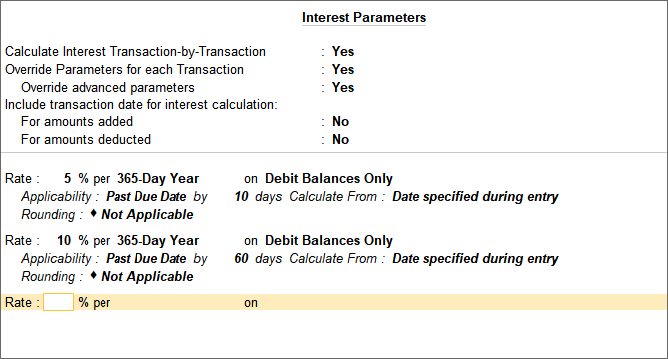13. Press Ctrl+A to accept the configuration.
14. Press Ctrl+A to save the ledger.

In case of the above configuration, interest will be calculated at 5% starting from 10th day after due date and at 10% starting from 60th day after due date. Similarly, multiple slabs can be configured with different interest style, applicability, calculate from parameter and round off value.

### Set advanced interest parameters for bank ledgers

1. In the Interest Parameters screen of bank ledger, select Bank/Reco Date to Calculate Interest Based on.
2. Enter the Rate, and Interest Style as required.
3. Enter the applicability period.
4. Press Enter and enter the interest calculation details for another period. The Interest Parameters screen appears as shown below: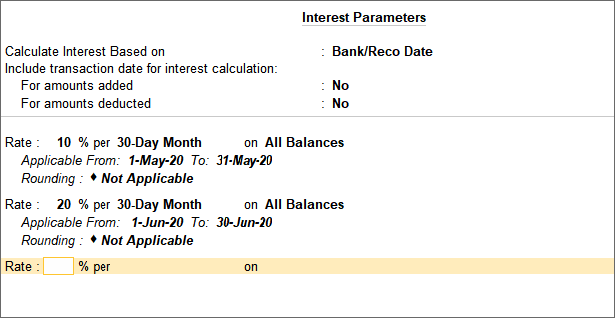5. Press Enter.
6. Press Ctrl+A to save the ledger.

## View Interest for a Group

The Group Interest Calculation statement is a list of all accounts and sub-groups belonging to the selected group. It displays the current balance in each account or sub-group, and the total interest.

1. Alt+G (Go To) > type or select Group Interest Calculation.
Alternatively, Gateway of Tally > Display More Reports > Statements of Accounts > Interest Calculations > Group.
2. Select a Group, for example, Current Liabilities.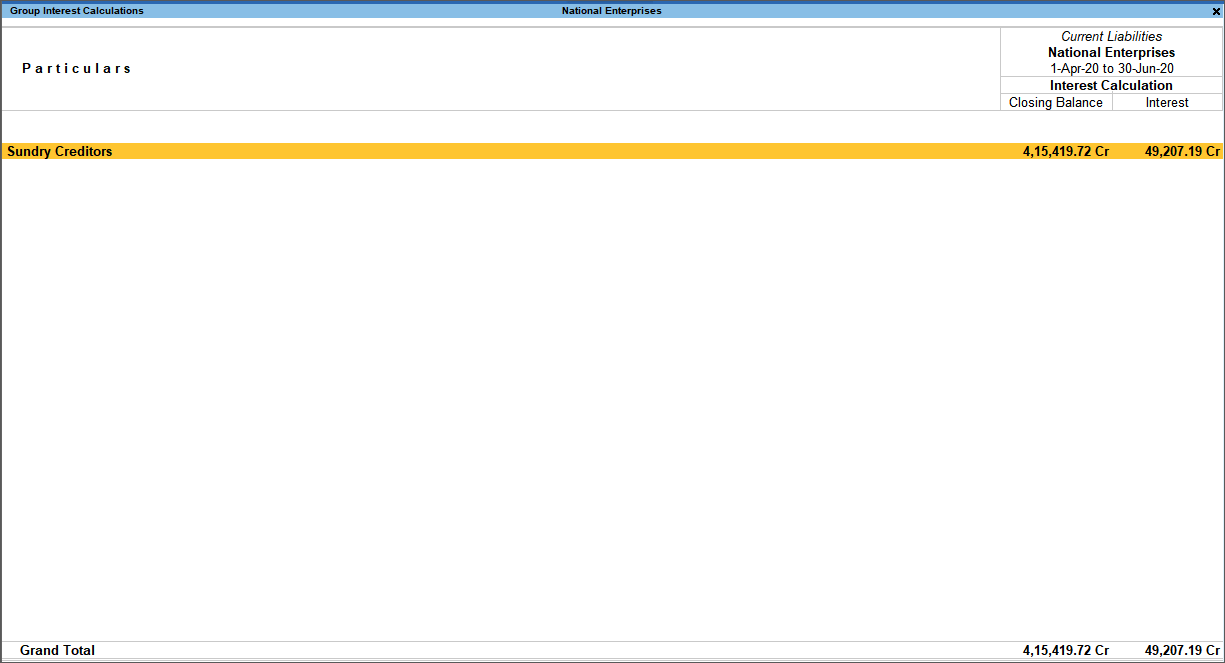`Note: The Cr in the interest amount indicates that it is a liability.`
3. Press Enter to drill down to the Ledger Interest report.

## View Interest on Ledger Balances

You can get a report on the ledger balances for the interest amount.

1. Alt+G (Go To) > type or select Ledger Interest Calculation.
Alternatively, Gateway of Tally > Display More Reports > Statements of Accounts > Interest Calculations > Ledger.
2. Select an account. The Ledger Interest report appears as shown below: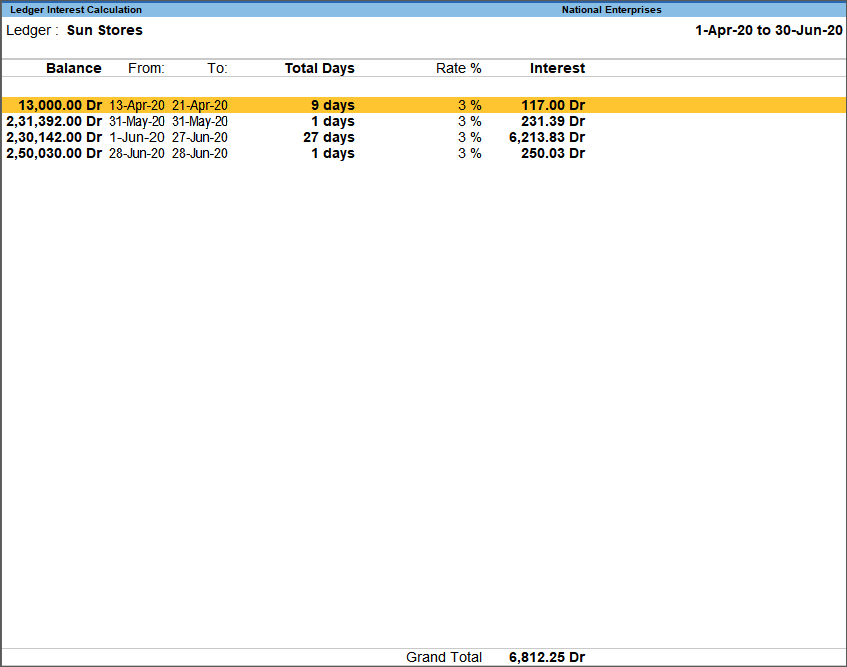## View Interest on Invoices

You can calculate interest on each invoice for the outstanding period. Transaction by transaction or bill-by-bill interest can be calculated for party ledgers (grouped under Sundry Debtors and Sundry Creditors). For calculating interest on each bill, the bill-wise details should be enabled for the company and the party.

The Ledger Interest report displays the interest calculated for the selected ledger. If a part of the amount is settled, then interest is calculated only on the amount pending to be repaid.

1. Alt+G (Go To) > type or select Ledger Interest Calculation> select the Ledger.
Alternatively, Gateway of Tally > Display More Reports > Statements of Accounts > Interest Calculations > Ledger.
2. Press Ctrl+H (Change View) > select Bill-wise Details > and press Enter.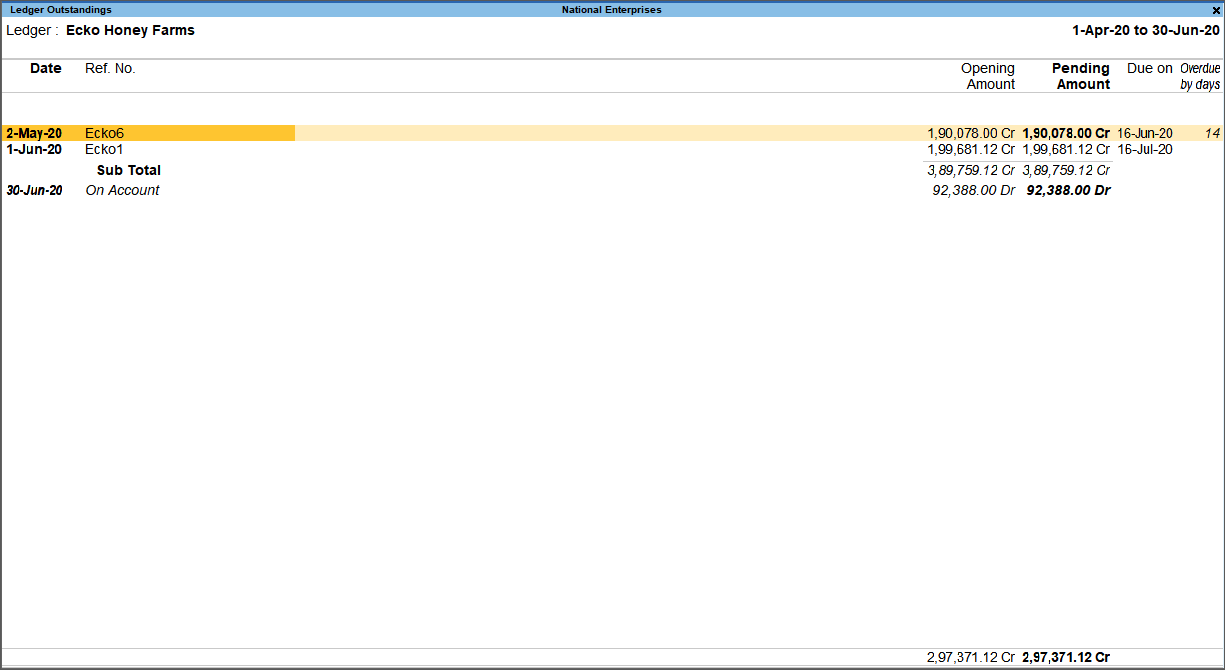3. Press Alt+F5 (Detailed) to view the calculation and the period.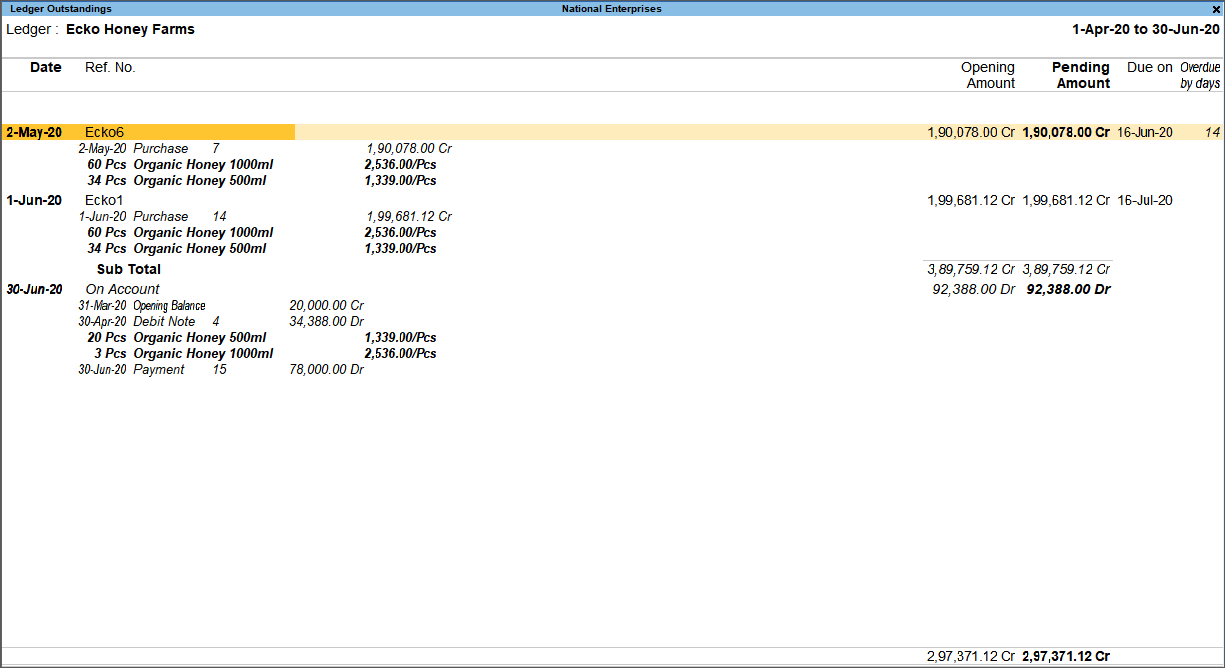`Note: If part of the amount is settled, the interest gets calculated on the remaining balance. Now check the interest amount due.`
`Note: The Cr in the interest amount indicates that it is a liability.`

The interest liability and expense can be accounted for by recording a Debit Note and Credit Note with voucher classes.

## Accounting for Interest Payable and Receivable

It is recommended to track the interest by using the Debit Note and Credit Note with voucher classes.

When you set the parameters for interest calculation, TallyPrime will only show the calculated interest value. However, the impact on the outstanding will be visible when a transaction is recorded to account for or book the expense. This is done by using a Credit Note with voucher class for interest payables or a Debit Note with voucher class for interest receivables.

If you do not want any interest tracking and would like to make a standard entry for accounting your interest liability and expenses, you can pass a Journal voucher entry.

### Use Voucher Classes for Interest Payable & Receivable

When you are paying interest for the loans taken use credit notes with voucher class for accounting the interest to be paid to the lender. When you record a credit note with voucher class, the amount is adjusted against the interest payable to the lender.

In a similar way when you have to receive interest for the loans you have given, use debit notes with voucher class.

Note: Alternatively, you can do manual entries to account for the interest payments and receipts. But this will not be adjusted against the interest amount.

#### Create Voucher Classes for Credit Note or Debit Note

1. Gateway of Tally > Alter > type or select Voucher Type > Credit Note > and press Enter.
Alternatively, Alt+G(Go to) > Alter Master > type or select Voucher Type > Credit Note > and press Enter
2. Enter the Name of Class. If you do not see this option use F12 (Configure) to get it.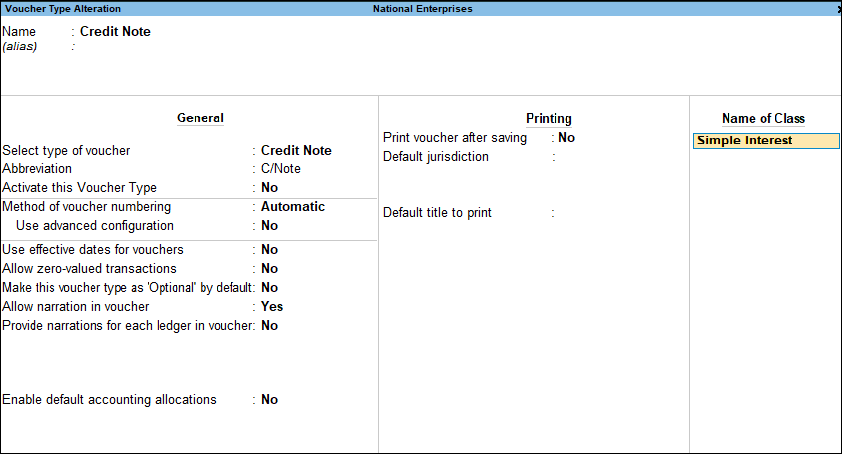3. Set up interest calculation: Simple interest.

4. Set up interest calculation: Compound interest.

#### Record Interest Payable or Receivable Using Voucher Class

Using voucher class for a sample credit note with interest is as follows.

1. Gateway of Tally > Vouchers> press F10 (Other Vouchers) > type or select Credit Note.
Alternatively, press Alt+G (Go To) >Create Voucher > press F10 (Other Vouchers) > type or select Credit Note.
In case the voucher is inactive, you will be prompted to activate the Credit Note voucher type. Select Yes or press Y to proceed.
2. Select the voucher class.
3. Select the ledgers to which you need to account the interest.
4. Credit ledger: Ledgers in which interest calculation is enabled are listed for selection.
5. Amount: The amount is calculated and displayed based on the voucher date.
6. Debit ledger: Select the appropriate ledger to debit.
7. Complete the entries, Accept the voucher screen. As always, you can press Ctrl+A to save.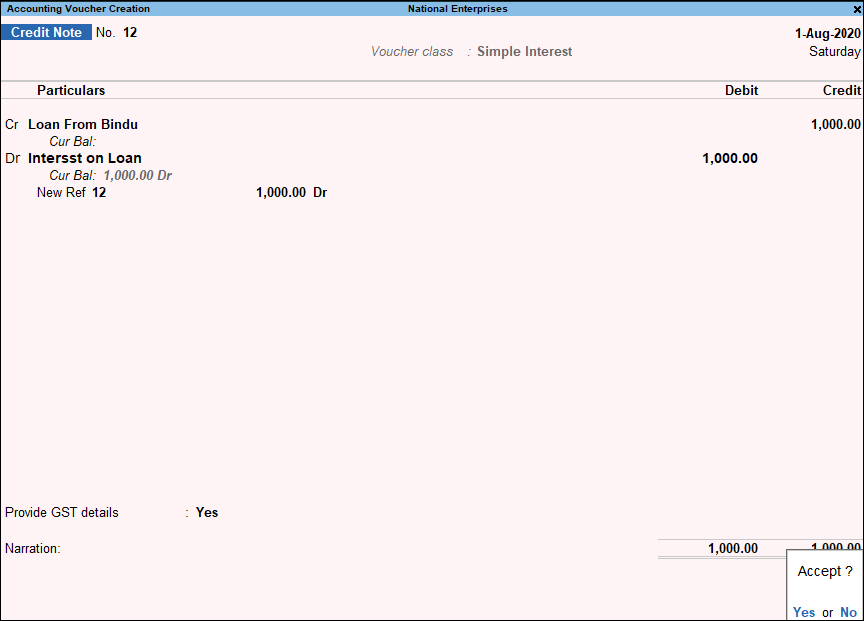In a similar way, you can create debit notes using voucher class.

### Record Credit Note with Voucher Class

Assume that you have borrowed Rs. 30,000 rupees from a third party, and you have recorded a Receipt entry to account for the loan amount received. If you want to know how much interest you have been accumulating on the loan amount on a daily basis with the rate of interest involved, you can refer to the Interest Calculation report.

The Interest Calculation report appears as shown below: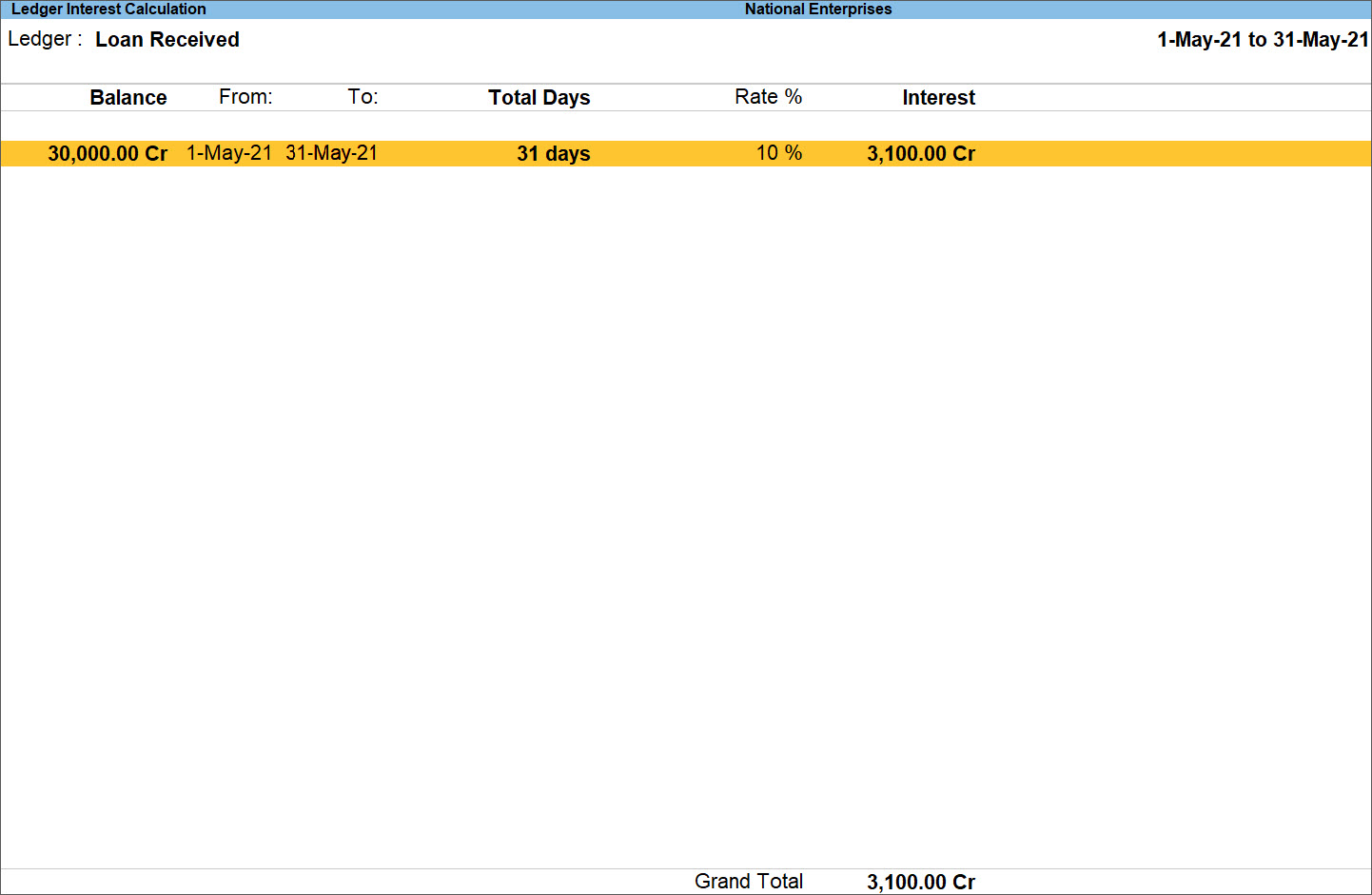The report shows you the amount of interest you will pay on the loan based on the date you choose. However, the impact on the outstanding will be visible in the report when you account for Payables by recording a Credit Note with voucher class.

When you record a Credit Note for the interest payable, any interest you incur on the loan amount will be determined by the date you choose. TallyPrime will pull the calculated value from the report and will display the final amount to pay.

1. Gateway of Tally > Vouchers > press F10 (Other Vouchers) > type or select Credit Note.
Alternatively, press Alt+G (Go To) > Create Voucher > press F10 (Other Vouchers) > type or select Credit Note.
In case the voucher is inactive, you will be prompted to activate the Credit Note voucher type. Select Yes or press Y to proceed.
2. Select the Voucher Class for Credit Note.
3. Press F2 (Date) to change the Voucher Date so that the interest can be calculated accordingly.
4. Enter the Particulars.
1. Credit ledger: Select the ledger to which the interest needs to be credited.
The interest amount will be automatically calculated based on the voucher date and displayed under the Credit field.
`Note: While recording the voucher, TallyPrime pulls the calculated interest value from the report and displays the amount to pay. `
2. Debit ledger: Select the expense ledger. You can create an expense ledger for the interest payable. The amount will be displayed automatically under Debit field.
5. Enter the other details, as applicable.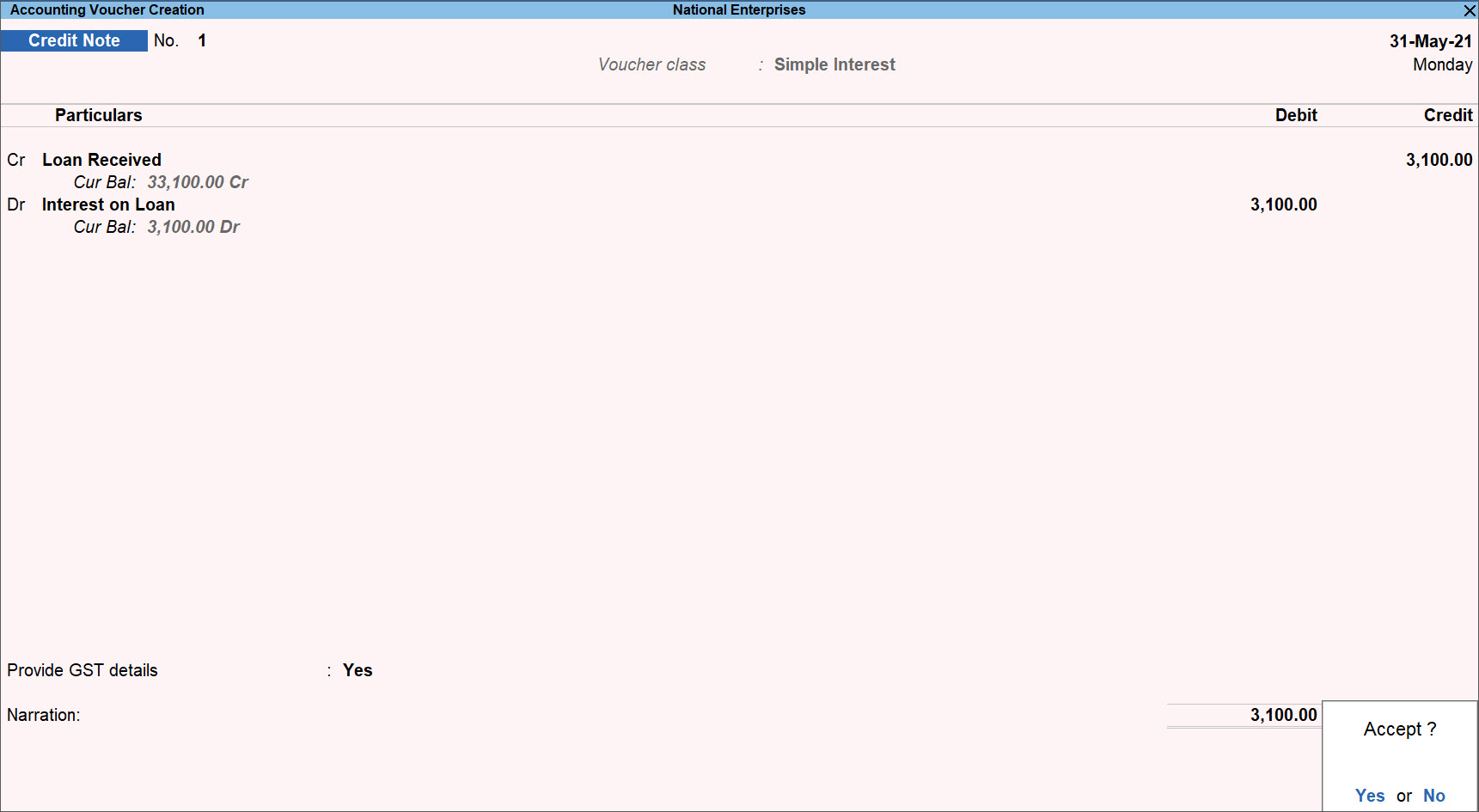An interest amount of Rs. 3100 is calculated based on the date you have selected. This amount is added to the loan sum and becomes your payable amount on that date.
6. Press Y to accept the screen. As always, you can press Ctrl+A to save.
In a similar way, you can record a Debit Note with voucher class for interest receivable.
With each payment on your loan, the balance amount will appear in the report on which the interest will be calculated based on the selected parameters and the date you choose to pay.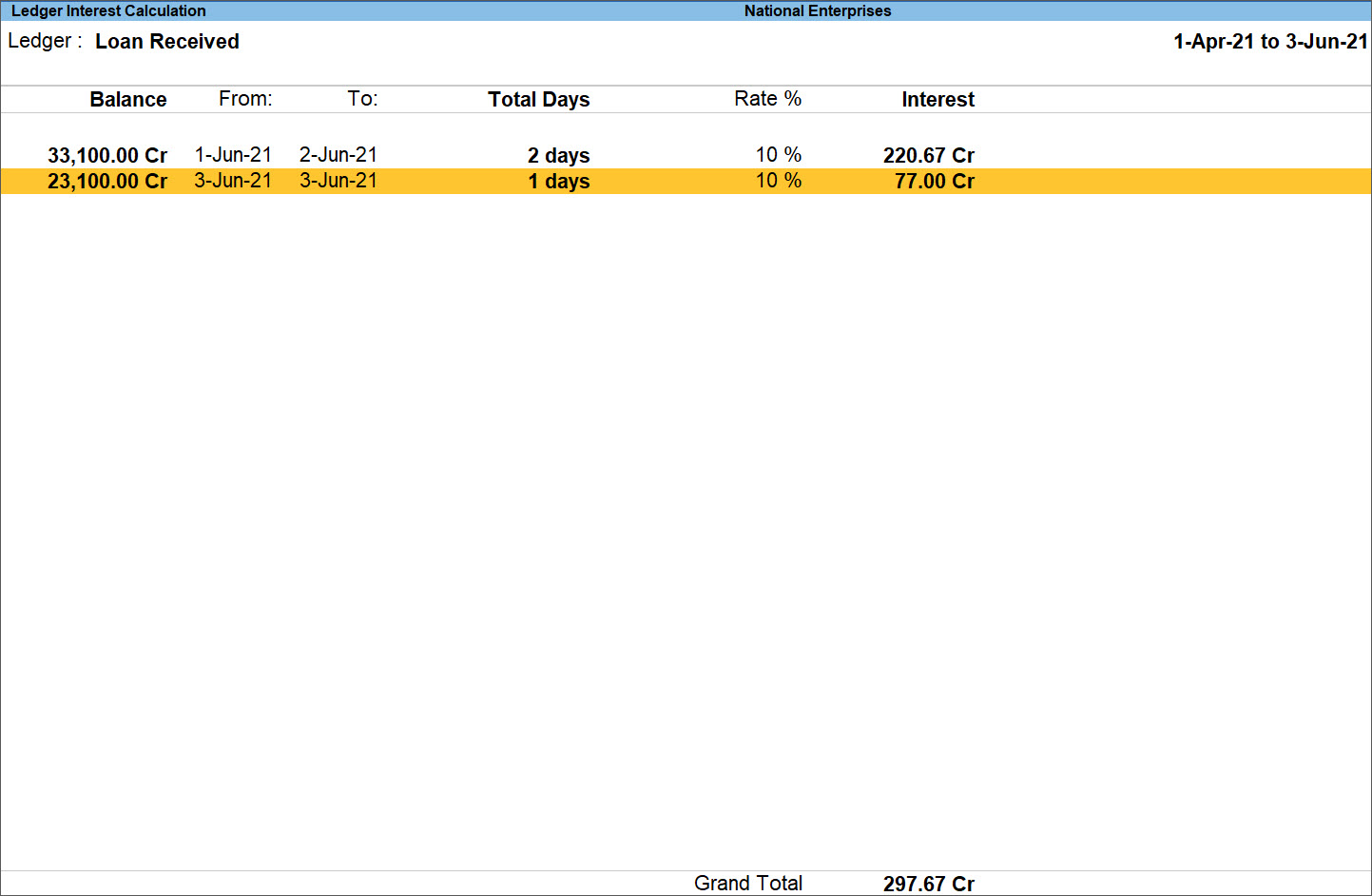In this way, Interest Payable can be recorded by passing a Credit Note with a voucher class and Interest Receivable by passing a Debit Note with a voucher class. As part of the repayment of interest, you can record a Payment voucher and a Receipt voucher as required under the loan terms.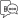Helpful?